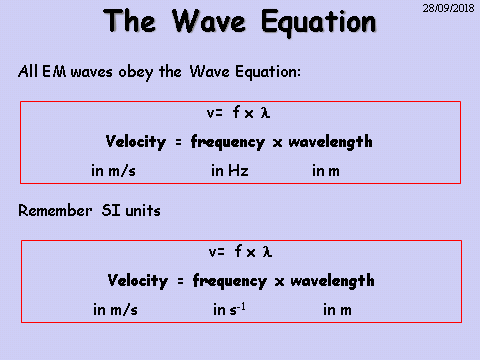# Properties of Waves

## Frequency

The frequency of a wave is the number of waves that passes a given point in one second. It is measured in Hertz (Hz). The equation for frequency is: Frequency = 1 / Time Period f = 1 / T

## Wave SpeedA water wave has a wavelength of 1m and a frequency of 2 Hz. How fast is the wave travelling?